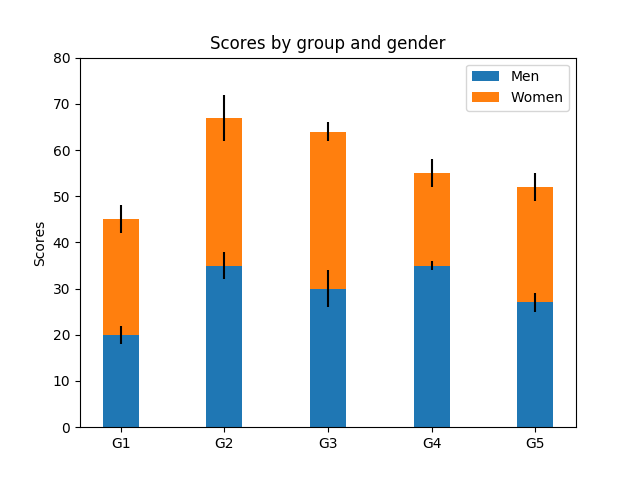Travis-CI:# Stacked Bar Graph¶

This is an example of creating a stacked bar plot with error bars using `bar`. Note the parameters yerr used for error bars, and bottom to stack the women’s bars on top of the men’s bars.```import numpy as np
import matplotlib.pyplot as plt

N = 5
menMeans = (20, 35, 30, 35, 27)
womenMeans = (25, 32, 34, 20, 25)
menStd = (2, 3, 4, 1, 2)
womenStd = (3, 5, 2, 3, 3)
ind = np.arange(N)    # the x locations for the groups
width = 0.35       # the width of the bars: can also be len(x) sequence

p1 = plt.bar(ind, menMeans, width, yerr=menStd)
p2 = plt.bar(ind, womenMeans, width,
bottom=menMeans, yerr=womenStd)

plt.ylabel('Scores')
plt.title('Scores by group and gender')
plt.xticks(ind, ('G1', 'G2', 'G3', 'G4', 'G5'))
plt.yticks(np.arange(0, 81, 10))
plt.legend((p1, p2), ('Men', 'Women'))

plt.show()
```

Total running time of the script: ( 0 minutes 0.030 seconds)

Gallery generated by Sphinx-Gallery